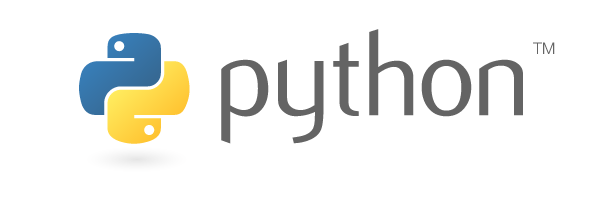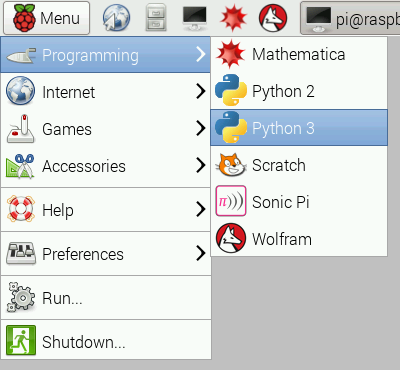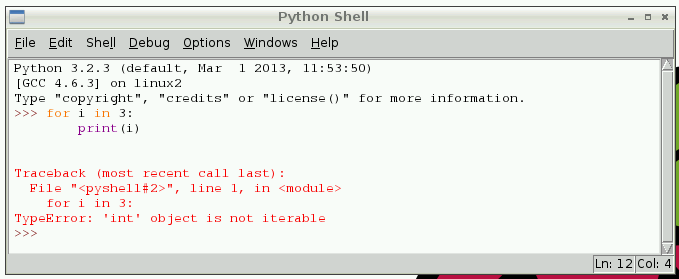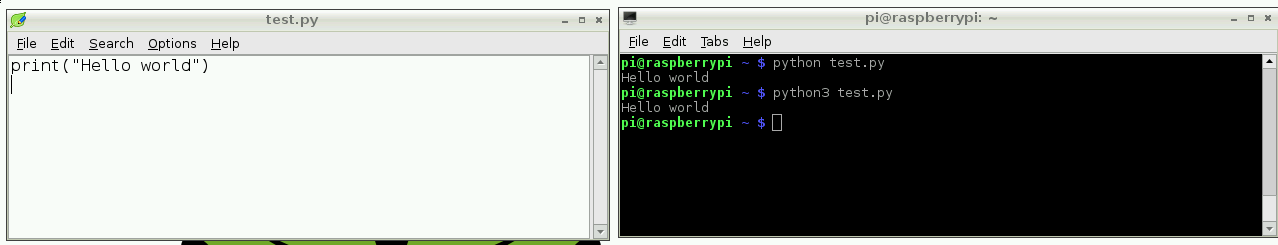# [原创翻译]Python（树莓派官方文档）

Python是一种美妙而强大的编程语言，易于读、写。在树莓派还可以让你的项目连接到现实世界。Python的语法非常干净，使用标准的英文关键字并强调可读性。从桌面上打开IDLE启动Python开发环境。

## IDLEIDLE通过REPL（阅读-执行-打印-循环）来提示你输入的Python命令。由于REPL的存在，你可以不使用`print`函数的情况下看到命令的输出。

``````>>> 1 + 2
3
>>> name = "Sarah"
>>> "Hello " + name
'Hello Sarah'``````

IDLE自带语法高亮和自动代码补全功能。使用快捷键 `Alt + P` (向前) 和 `Alt + N` (向后)可以在REPL中查看输入命令的历史纪录。

## Python 基本用法

Python中的Hello world：

``print("Hello world")``

### 缩进

``````for i in range(10):
print("Hello")``````

``````for i in range(2):
print("A")
print("B")``````

``````A
B
A
B``````

``````for i in range(2):
print("A")
print("B")``````

``````A
A
B``````

### 变量

``````name = "Bob"
age = 15``````

``````age = 15
age += 1  # increment age by 1
print(age)``````

### 注释

``````"""
This is a very simple Python program that prints "Hello".
That's all it does.
"""

print("Hello")``````

### 列表

Python中的列表（在某些语言中称为数组）是可以是任意类型数据的集合：

``numbers = [1, 2, 3]``

### 迭代

``````numbers = [1, 2, 3]

for number in numbers:
print(number)``````

`numbers`指向每次遍历的列表项，然后打印出来：

``````1
2
3``````

``````dog_name = "BINGO"

for char in dog_name:
print(char)``````

``````B
I
N
G
O``````

### 范围

``````for i in 3:
print(i)``````

``TypeError: 'int' object is not iterable````````for i in range(3):
print(i)``````

`range(5)` 包含 `0``1``2``3``4` (共5个数字). 要获取`1``5` 使用`range(1, 6)`

### 长度

``````name = "Jamie"
print(len(name))  # 5

names = ["Bob", "Jane", "James", "Alice"]
print(len(names))  # 4``````

### If 控制语句

`if`条件判断的用法如下：

``````name = "Joe"

if len(name) > 3:
print("Nice name,")
print(name)
else:
print("That's a short name,")
print(name)``````

## IDLE中的Python文件

IDLE中点击`File > New File`来新建Python文件，你会看到一个空白的窗口。这是一个空文件，而不是之前我们使用的交互窗口。你可以在这里编写Python代码，然后保存它并运行，但输出结果将在另一个窗口中展示。

``````n = 0

for i in range(1, 101):
n += i

print("The sum of the numbers 1 to 100 is:")
print(n)``````

## 从命令行执行Python文件## More

• Python 2 vs. Python 3
• 公共约定
• Python其它用法
• 安装 Python 库
• GPIO